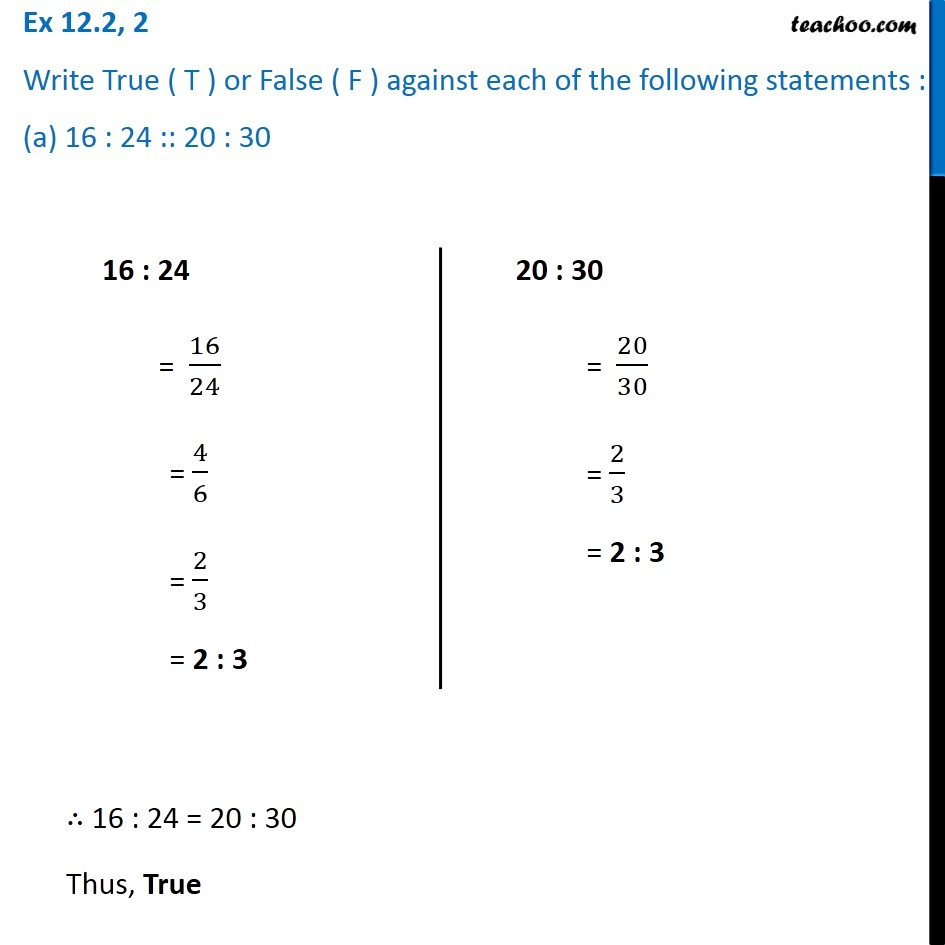Proportion

Chapter 12 Class 6 Ratio and Proportion
Concept wiseLearn in your speed, with individual attention - Teachoo Maths 1-on-1 Class

### Transcript

Ex 12.2, 2 Write True ( T ) or False ( F ) against each of the following statements : (a) 16 : 24 :: 20 : 30 16 : 24 = 16/24 = 4/6 = 2/3 = 2 : 3 20 : 30 = 20/30 = 2/3 = 2 : 3 ∴ 16 : 24 = 20 : 3021 : 6 = 21/6 = 7/2 = 7 : 2 Thus, True